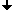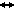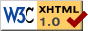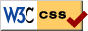Nonlinear Dynamics at the Free University Berlin

Summer 2013

# Seminar Advanced Topics in Nonlinear Dynamics

Prof. Dr. Bernold Fiedler, PD Dr. P. Gurevich, Dr. Stefan Liebscher

## Program

 Apr 11, 2013 Sina Reichelt(WIAS Berlin) Introduction to concepts in homogenization theory The fundamental aim of homogenization is to derive from an inhomogeneous problem, which is for instance depending on different spatial scales or on microstructures, a simpler "effective" model problem. The effective problem (which is only sometimes homogeneous) is expected to preserve the characteristic properties of the original problem, but is usually easier to handle - analytically or numerically. In this talk, I will introduce different concepts in the theory of homogenization by studying an elliptic prototype problem. Finally I will outline how those concepts are applicable to nonlinear reaction-diffusion equations. Apr 18, 2013 Juliette Hell(Free University Berlin) Dynamics at infinity for slowly nondissipative reaction-diffusion-equation with a jumping nonlinearity Apr 25, 2013 Marek Fila(Comenius University, Bratislava) Non-accessible singular homoclinic orbits for a semilinear parabolic equation We show the existence of at least three different continuations beyond blow-up for a backward self-similar solution of a supercritical Fujita equation. One of these extended solutions cannot be approximated by classical entire solutions in a specific way given by the scaling invariance of the equation, while the minimal continuation is known to be accessible by a family of such entire solutions. May 2, 2013 Claes Uggla(Karlstad University, Sweden) Some aspects concerning ODE problems in Cosmology and Astrophysics In this talk I'll review some general features of ODE problems that arise in cosmology and astrophysics within the context of General Relativity. In particular, I'll first describe why and how a common hierarchical structure arises and which all problems in this area share. As a consequence it is necessary to understand these structures, especially those at the bottom of the hierarchy, in order to be able to derive any results concerning models higher up in the hierarchy, e.g., models with so-called oscillatory singularities. To be more specific, I'll then present very simple examples that describe the key elements, and I will show how they can be tackled with various techniques, step by step increasing the complexity. I'll finally conclude with some comments about how these ODE problems then fit into a generic PDE context of general relativity. May 16, 2013 Arnd Scheel(University of Minnesota, School of Mathematics) Pattern selection in the wake of fronts May 30, 2013 Bernhard Brehm(Free University Berlin) Analytic estimates on some Poincare-maps for Bianchi 9 heteroclinics In Bianchi 8 and 9 cosmologies, estimates for the transit near the Kasner circle of equilibria are essential for questions regarding long-time dynamics. In the fall 2012 and January 2013, I presented new estimates on the transit in a complexified version of the Bianchi differential equations. This talk will focus on proving at least parts of these estimates rigorously (since previous attempts at presenting the technical details were derailed by small mistakes in my proof and a less-than-clear organisation of my estimates colliding with the usual Thursday crowd). The talk should hopefully be mostly understandable even without knowing Bianchi or remembering my previous talk on this topic. Jun 6, 2013 Eyal Ron(Free University Berlin) Controlling the controller: differential equations with hysteresis and delay Our talk revolves around differential equations with hysteresis and delay terms. We focus on the problem of stability analysis of periodic solutions of such equations. This problem is infinite dimensional in nature due to the delay. We present a technique to reduce it, in certain cases, to a finite dimensional problem. Our main application is a thermal control model. It consists of a parabolic equation with hysteresis on the boundary. Gurevich and Tikhomirov showed recently the existence of both stable and unstable periodic solutions for such a model. Their result naturally raises the question of whether it is possible to change the stability properties of such solutions. We use the well-known Pyragas control to change the stability of periodic solutions of the thermal control model. Using this method, one adds an additional delay term to the boundary without destroying the known periodic solution. This results in a parabolic equation with both hysteresis and delay terms on the boundary. Using Fourier decomposition, this equation is reduced to a system of ODE. Then we can apply our finite dimensional reduction technique and show that Pyragas control can change stability of periodic solutions. Jun 13, 2013 Hayato Chiba(University of Kyushu, Japan) A spectral theory of linear operators on a Gelfand triplet and its application to coupled oscillators Dynamics of systems of large populations of coupled oscillators have been of great interest because collective synchronization phenomena are observed in a variety of areas. The Kuramoto model is often used to investigate such phenomena. In this talk, an infinite dimensional Kuramoto model is considered, and Kuramoto's conjecture on a bifurcation diagram of the system will be proved. For this purpose, a new spectral theory of linear operators based on a Gelfand triplet is established. Jun 20, 2013 Atsushi Mochizuki(RIKEN, Tokyo) A brief invitation to gene regulation Jun 27, 2013 Joa Weber(Bielefeld University, Berlin) A backward lambda-lemma for the forward heat flow Fix a closed Riemannian manifold M and consider the classical action functional given on a loop in M by integrating kinetic minus potential energy along the loop. Here we assume that the potential V is a time dependent function on M such that the action is a Morse functional. Then the downward L2-gradient of the action generates an infinite dimensional hyperbolic dynamical system on the loop space of M, namely the well known (forward) heat semi-flow. Its fixed points are the critical points of the action and these are the (perturbed) closed geodesics in M. A fundamental tool in finite dimensional dynamical systems is the lambda-Lemma which asserts that any disk transversal to the unstable manifold of a hyperbolic fixed point and of complementary dimension C1-converges under the backward flow locally to the stable manifold. We prove an analogue for the heat flow although there is no backward flow. The first application is a construction of a Morse filtration of the loop space which has the property that the boundary operator of the associated cellular complex is given by counting downward heat flow lines with signs. Since the cellular chain groups are generated by the critical points of the action and graded by the Morse index we obtain a natural isomorphism between singular homology of the loop space and Morse homology associated to the downward L2-gradient of the action functional. Jun 27, 2013 Bernold Fiedler(Free University Berlin, Berlin) ODE meanders and PDE global attractors: a Sturmian view For scalar parabolic equations in one space dimension there are many results which relate the equilibrium ODE v" + f(x,v,v') = 0 with the global structure of the PDE attractor of the associated semilinear parabolic ODE, say under Neuman boundary conditions on the unit interval. A central notion is the meander permutation introduced by Fusco and Rocha in 1991. If f is 1-periodic in x, transverse homoclinic orbits may arise for the above "pendulum". On intervals (0,L) of large integer length L, these homoclinics strongly influence the behavior of the associated meander permutation, and hence of the PDE attractor. Although the precise effect remains elusive, at present, we attempt to illustrate the problem in the more elementary case of standard Anosov diffeomorphisms on the 2-torus. Jul 4, 2013 Jul 11, 2013 Bachelor students(Free University Berlin) Ongoing research

## Time and Place

Talks usually take place on Thursday at 2:15 p.m.
at the Free University Berlin
Room 130, Arnimallee 3 (rear building), 14195 Berlin.

Guests are always welcome!

## ArchiveLast change: Jun. 25, 2013
 This page strictly conforms to the XHTML1.0 standard and uses style sheets.